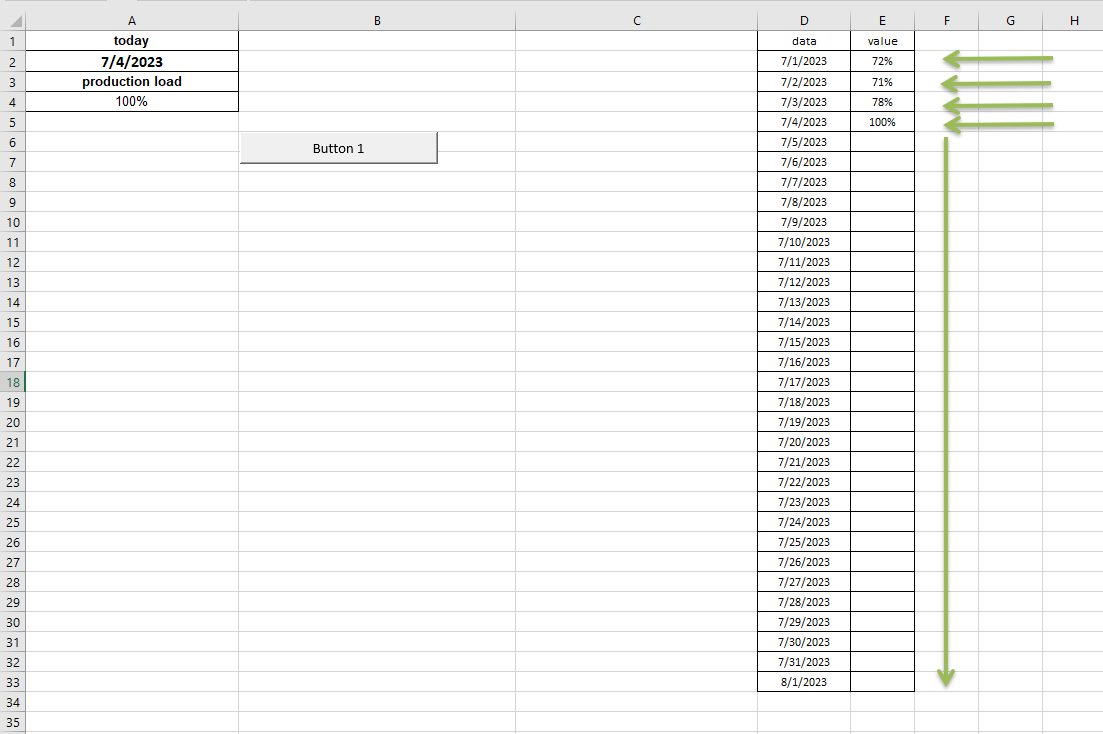# Transforming a Microsoft Office macro into an ONLYOFFICE macro

6 July 2023

Have you ever wondered how to convert your Microsoft Office VBA macros into macros that work seamlessly with ONLYOFFICE? While there’s no direct solution to this conversion, in this article, we will walk you through the steps of transforming a VBA macro that was sent to us by one of our users.## VBA macro

Here’s the original VBA macro:

``````Sub Button1_Click()
Dim mycel As Range
For Each mycel In Columns("D:D").SpecialCells(xlCellTypeConstants, 23)
If mycel = [TODAY()] Then mycel.Offset(0, 1) = [This_value]
Next
End Sub
``````

This macro scans column D for cells with a constant value of 23 and checks if each cell’s value is equal to the value in the cell labeled [TODAY(]. If the values match, it updates the cell in the next column, offset by one column to the right, with the value in the cell labeled [This_value].## Building the ONLYOFFICE macro

To convert this macro into a JavaScript macro compatible with ONLYOFFICE, we can leverage the ONLYOFFICE API.

In the ONLYOFFICE macro, we start by obtaining the active sheet using the Api.GetActiveSheet() function. We then retrieve the values from cells A2 and A4, which correspond to [TODAY()] and [This_value] in the VBA macro:

``````var sheet = Api.GetActiveSheet();
var dateValue = sheet.GetRange("A2").GetValue();
var updateValue = sheet.GetRange("A4").GetValue();
``````

Next, we define the range of cells we want to iterate over using sheet.GetRange(“D1:D10”). This range represents column D in this example, but you can adjust it according to your specific needs:

``````var dateRange = sheet.GetRange("D1:D10");
``````

We use the ForEach function to iterate over each cell in the defined range. For each cell, we retrieve the current value using range.GetValue() and compare it to the value in cell A2. If they match, we get the row number of the current cell using range.GetRow(), and then obtain the corresponding cell in column E using sheet.GetRange(“E” + row):

``````dateRange.ForEach(function(range) {
var currentDate = range.GetValue();
// Check if the current date matches the value in cell A2
if (currentDate === dateValue) {
// Get the corresponding cell in column E
var row = range.GetRow();
var oRange = sheet.GetRange("E" + row);  }
});
``````

Finally, we update the value in the next cell (offset by one column to the right) with the value from cell A4 using oRange.SetValue(updateValue):

``````   // Update the value in the next cell
oRange.SetValue(updateValue);
}
});
``````

The entire macro code is the following:

``````(function()
{
var sheet = Api.GetActiveSheet();
var dateValue = sheet.GetRange("A2").GetValue();
var updateValue = sheet.GetRange("A4").GetValue();
var dateRange = sheet.GetRange("D1:D10");
dateRange.ForEach(function(range) {
var currentDate = range.GetValue();
// Check if the current date matches the value in cell A2
if (currentDate === dateValue) {
// Get the corresponding cell in column E
var row = range.GetRow();
var oRange = sheet.GetRange("E" + row);
// Update the value in the next cell
oRange.SetValue(updateValue);
}
});
})();
``````

Let’s run the macro and see how the macro works!

In conclusion, there is no direct conversion from VBA macros to JavaScript macros for ONLYOFFICE. However, you can use your existing VBA macros as a reference when building macros for ONLYOFFICE. By leveraging the ONLYOFFICE API methods and making the necessary adjustments, you can achieve similar functionality.

While it may require some code restructuring, the process is manageable. We hope that with some guidance outlined in the article, you can successfully create powerful ONLYOFFICE macros. Embrace the possibilities, enjoy the benefits, and best of luck in your exploratory endeavors!